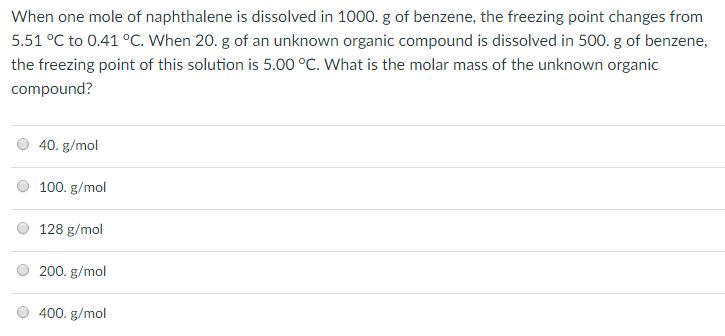# Question & Answer: When one mole of naphthalene is dissolved in 1000. g of benzene, the freezing point changes…..Don't use plagiarized sources. Get Your Custom Essay on
Question & Answer: When one mole of naphthalene is dissolved in 1000. g of benzene, the freezing point changes…..
GET AN ESSAY WRITTEN FOR YOU FROM AS LOW AS \$13/PAGE

When one mole of naphthalene is dissolved in 1000. g of benzene, the freezing point changes from 5.51 degree C to 0.41 degree C. When 20. g of an unknown organic compound is dissolved in 500. g of benzene, the freezing point of this solution is 5.00 degree C. What is the molar mass of the unknown organic compound? 40. g/mol 100. g/mol 128 g/mol 200. g/mol 400. g/mol

We know depression in freezing point is given by

where  is depression in freezing point

Kf is ebuilliscopic constant

and m is molality of substance

Given intially when one mole of napthalene is dissolved in 1 kg of benzene solution

so

m=1/1=1 m

therefore kf=5.1

In next case let molecualr weight of organic compound be M

So moles of it=20/M

now mass of benzene=0.5 kg

molality=m=20/M*0.5=40/M

now Kf=5.1 and

so 0.51=40/M*5.1

thus M=400 g